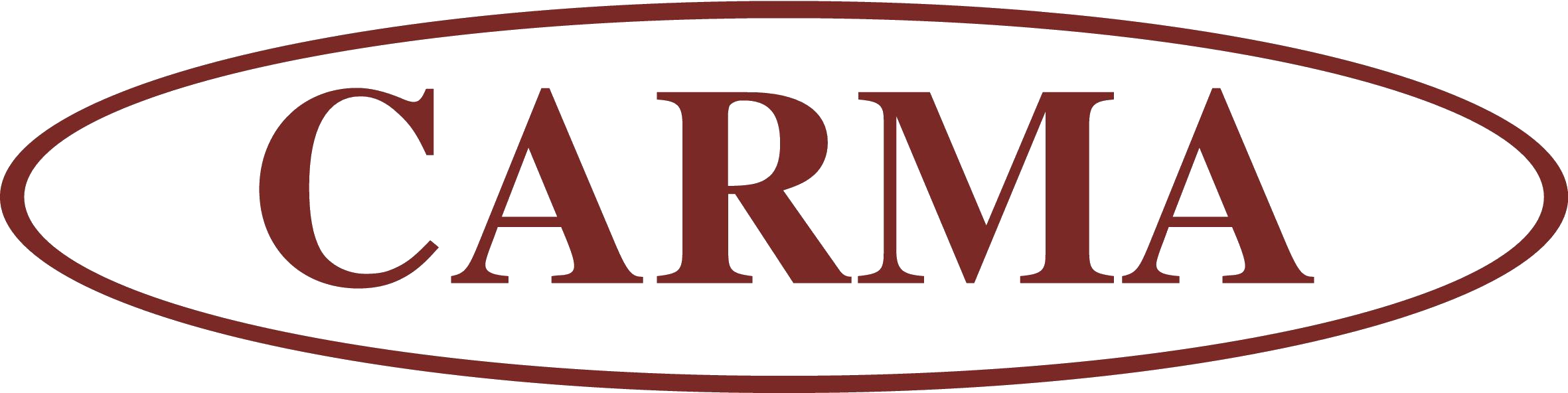# SIGMAopt Seminar

## Wednesday, 11th Apr 2012

V205, Mathematics Building

(Rescheduled from 10th April)

# Dr Jean Lasserre

(LAAS-CNRS, Université de Toulouse)

# Sublevel sets of positively homogeneous functions and non-Gaussian integrals

We investigate various properties of the sublevel set $\{x : g(x) \leq 1\}$ and the integration of $h$ on this sublevel set when $g$ and $h$ are positively homogeneous functions. For instance, the latter integral reduces to integrating $h\exp(- g)$ on the whole space $\mathbb{R}^n$ (a non-Gaussian integral) and when $g$ is a polynomial, then the volume of the sublevel set is a convex function of its coefficients.

In fact, whenever $h$ is non-negative, the functional $\int \phi(g)h dx$ is a convex function of $g$ for a large class of functions $\phi:\mathbb{R}_{+} \to \mathbb{R}$. We also provide a numerical approximation scheme to compute the volume or integrate $h$ (or, equivalently, to approximate the associated non-Gaussian integral). We also show that finding the sublevel set $\{x : g(x) \leq 1\}$ of minimum volume that contains some given subset $K$ is a (hard) convex optimization problem for which we also propose two convergent numerical schemes. Finally, we provide a Gaussian-like property of non-Gaussian integrals for homogeneous polynomials that are sums of squares and critical points of a specific function.